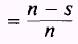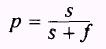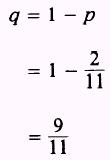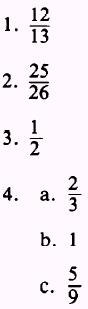Probability of FailureCustom SearchPROBABILITY OF FAILURE As before, if a trial results in any of n equally likely ways, and s is the number of successful ways and f is the number of failures, the probability of failure isorwhere s+f=n or n-s=f A trial must result in either success or failure. If success is cerŁtain then p equals 1 and q equals 0. If success is impossible then p equals 0 and q equals 1. Combining both events, for either case, makes the probability of success plus the probability of failure equal to 1. IfandthenIf, in any event p+q=1 thenIn the case of tossing a coin, the probability of success isand the probability of failure isEXAMPLE: What is the probability of not drawing a black marble from a box containing 6 white, 3 red, and 2 black marbles? SOLUTION: The probability of drawing a black marble from the box isSince the probability of drawing a marble is 1, then the probability of not drawing a black marble isPRACTICE PROBLEMS: Compare the following problems and answers with the preceding problems dealing with the probability of success: 1. What is the probability of not drawing an ace from a standard deck of 52 playing cards? 2. What is the probability of not drawing a black ace from a standard deck of playing cards? 3. If a die is rolled, what is the probability of an odd number not showing on the upper face? 4. A man has 3 nickels, 2 dimes, and 4 quarters in his pocket. If he draws a single coin from his pocket, what is the proŁbability that a. he will not draw a nickel? b. he will not draw a half-dollar? c. he will not draw a quarter? ANSWERS:Integrated Publishing, Inc. - A (SDVOSB) Service Disabled Veteran Owned Small Business